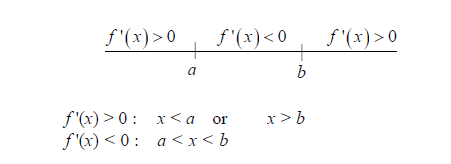# Examples on Maxima and Minima Set 1

Go back to  'Applications of Derivatives'

Example – 21

Find the extrema points of  $$f\left( x \right) = 3{x^4} - 4{x^3} - 36{x^2} + 28$$.

\begin{align}{\rm\bf{Solution:}} \qquad \qquad f'\left( x \right) &= 12{x^3} - 12{x^2} - 72x\\\\& = 12x\left( {{x^2} - x + 6} \right)\\\\ &= 12x\left( {x + 2} \right)\left( {x - 3} \right)\end{align}

We determine the sign of f '(x) using a number line:From the number line, observe that (using the FODT):

$$x = - 2\,\,{\rm{and}}\;x = 3$$  are local minima

$$x = 0$$ is a local maximum

Alternatively, we can use the SODT:

\begin{align}f''\left( x \right)& = 36{x^2} - 24x - 72\\&= 12\left( {3{x^2} - 2x - 6} \right)\\\\f''\left( 0 \right) < 0 \quad \Rightarrow \quad x &= 0 \text{ is a local maximum}\\\\f''\left( { - 2} \right) > 0 \quad \Rightarrow \quad x &= - 2 \text{ is a local minimum}\\\\f''\left( 3 \right) > 0 \quad \Rightarrow \quad x &= 3 \text{ is a local minimum.}\end{align}

Example – 22

Let $$f\left( x \right) = 2{x^3} - 3\left( {a + b} \right){x^2} + 6abx.$$  If a < b, determine the local maximum/minimum points of f (x). If a = b, how will the answer change?

\begin{align}{\rm\bf{Solution:}}\qquad\qquad {f}'(x)&=6{{x}^{2}}-6(a+b)x+6ab\\&=6(x-a)(x-b)\end{align}

To determine the sign of f '(x) in different intervals, we use a number line:Observe that f '(x) changes from positive to negative in the neighbourhood of x = a.

$\Rightarrow \qquad x=a\,\text{is a point of local maximum}$

Similarly, f '(x) changes from negative to positive in the neighbourhood of x = b.

$\Rightarrow \qquad x=b\,\text{is a point of local minimum}.$

If a = b, $${\rm{f'(x) = 6 (x - a}}{{\rm{)}}^2}$$

Notice that f '(x) is never negative. f '(x) is always positive except at x = a where f '(x) = 0

$\Rightarrow \qquad x=a\text{ is a point of inflexion.}$